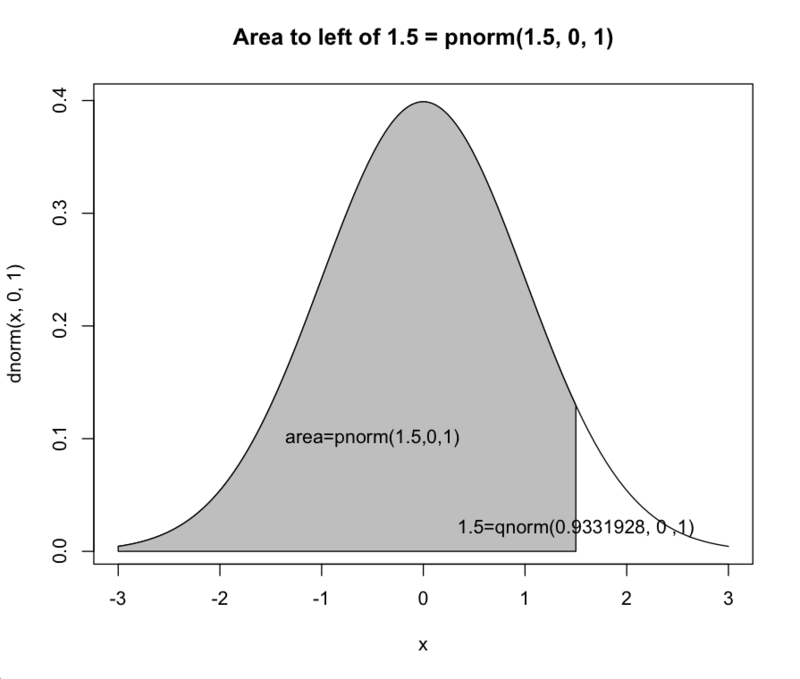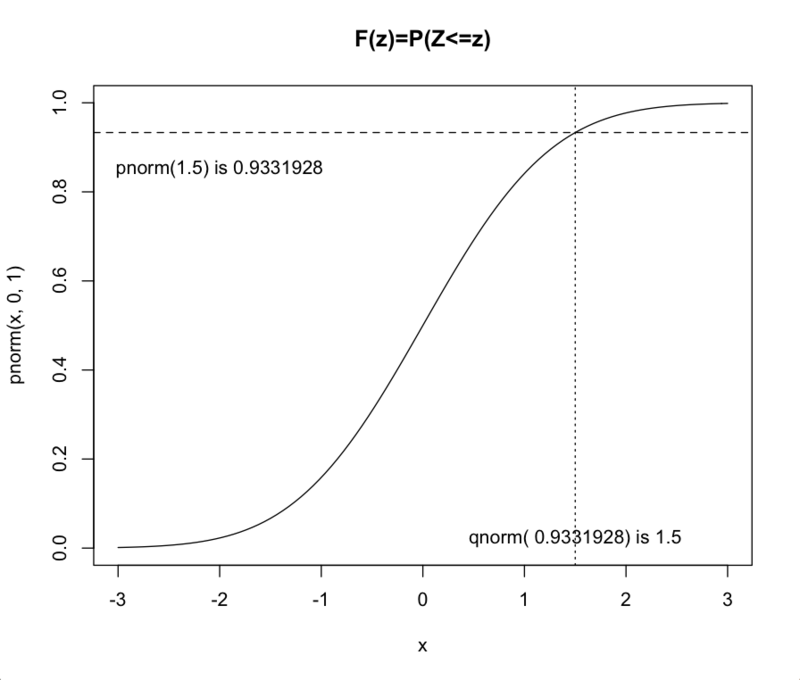# R軟體應用於機率密度曲線與累積分配曲線的繪製

> pnorm(1.5, 0, 1)

 0.9331928

> qnorm(0.9331928, 0, 1)

 1.5

> curve(dnorm(x, 0, 1), xlim = c(-3, 3), main = "Area to left of 1.5 = pnorm(1.5, 0, 1)")

> cord.x <- c(-3, seq(-3, 1.5, 0.01), 1.5)

> cord.y <- c(0, dnorm(seq(-3, 1.5, 0.01)), 0)

> polygon(cord.x, cord.y, col = "grey")

> text(-0.5, 0.1, "area=pnorm(1.5,0,1)")

>text(1.5, 0.02, "1.5=qnorm(0.9331928, 0 ,1)")> curve(pnorm(x, 0, 1), xlim = c(-3,3), main = "F(z)=P(Z<=z)")

> abline(h = 0.9331928, lty = 2)

> abline(v = 1.5, lty = 3)

> text(-2, 0.85, "pnorm(1.5) is 0.9331928")

> text(1.5, 0.02, "qnorm( 0.9331928) is 1.5")參考資料：

Verzani, J. (2001), simpleR &ndash; Using R for Introductory Statistics, The CSI MathDepartment, City University of New York.

 撰文者： 鄒慶士 博士 現任： 北商資訊與決策科學所教授 中華 R 軟體學會理事長 信箱：